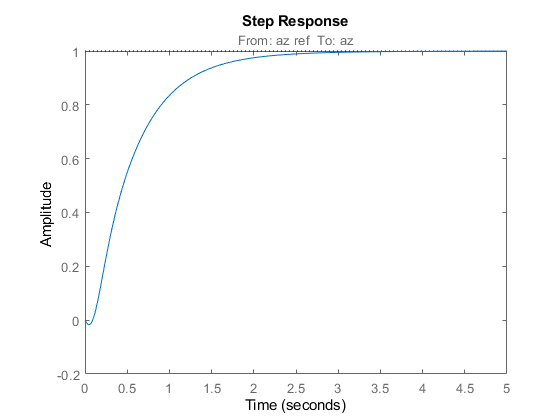Main Content

# Using Parallel Computing to Accelerate Tuning

This example shows how to leverage the Parallel Computing Toolbox™ to accelerate multi-start strategies for tuning fixed-structure control systems.

### Background

Both `systune` and `looptune` use local optimization methods for tuning the control architecture at hand. To mitigate the risk of ending up with a locally optimal but globally poor design, it is recommended to run several optimizations starting from different randomly generated initial points. If you have a multi-core machine or have access to distributed computing resources, you can significantly speed up this process using the Parallel Computing Toolbox.

This example shows how to parallelize the tuning of an airframe autopilot with `looptune`. See the example "Tuning of a Two-Loop Autopilot" for more details about this application of `looptune`.

### Autopilot Tuning

The airframe dynamics and autopilot are modeled in Simulink.

```open_system('rct_airframe1') ```The autopilot consists of two cascaded loops whose tunable elements include two PI controller gains ("az Control" block) and one gain in the pitch-rate loop ("q Gain" block). The vertical acceleration `az` should track the command `azref` with a 1 second response time. Use `slTuner` to configure this tuning task (see "Tuning of a Two-Loop Autopilot" example for details):

```ST0 = slTuner('rct_airframe1',{'az Control','q Gain'}); addPoint(ST0,{'az ref','delta fin','az','q'}) % Design requirements wc = [3,12]; % bandwidth TrackReq = TuningGoal.Tracking('az ref','az',1); % tracking ```

### Parallel Tuning with LOOPTUNE

We are ready to tune the autopilot gains with `looptune`. To minimize the risk of getting a poor-quality local minimum, run 30 optimizations starting from 30 randomly generated values of the three gains. Configure the `looptune` options to enable parallel processing of these 30 runs:

```rng('default') Options = looptuneOptions('RandomStart',30,'UseParallel',true); ```

Next call `looptune` to launch the tuning algorithm. The 30 runs are automatically distributed across available computing resources:

```Controls = 'delta fin'; Measurements = {'az','q'}; [ST,gam,Info] = looptune(ST0,Controls,Measurements,wc,TrackReq,Options); ```
```Starting parallel pool (parpool) using the 'local' profile ... connected to 6 workers. Final: Failed to enforce closed-loop stability (max Re(s) = 0.042) Final: Failed to enforce closed-loop stability (max Re(s) = 0.039) Final: Failed to enforce closed-loop stability (max Re(s) = 0.041) Final: Failed to enforce closed-loop stability (max Re(s) = 0.041) Final: Failed to enforce closed-loop stability (max Re(s) = 0.041) Final: Failed to enforce closed-loop stability (max Re(s) = 0.082) Final: Failed to enforce closed-loop stability (max Re(s) = 0.041) Final: Peak gain = 1.23, Iterations = 53 Final: Peak gain = 62, Iterations = 92 Some closed-loop poles are marginally stable (decay rate near 1e-07) Final: Peak gain = 62, Iterations = 128 Some closed-loop poles are marginally stable (decay rate near 1e-07) Final: Peak gain = 1.23, Iterations = 128 Final: Failed to enforce closed-loop stability (max Re(s) = 0.041) Final: Peak gain = 1.23, Iterations = 130 Final: Failed to enforce closed-loop stability (max Re(s) = 0.041) Final: Failed to enforce closed-loop stability (max Re(s) = 0.041) Final: Failed to enforce closed-loop stability (max Re(s) = 0.04) Final: Failed to enforce closed-loop stability (max Re(s) = 0.041) Final: Failed to enforce closed-loop stability (max Re(s) = 0.082) Final: Failed to enforce closed-loop stability (max Re(s) = 0.041) Final: Peak gain = 1.23, Iterations = 98 Final: Peak gain = 61.9, Iterations = 79 Final: Failed to enforce closed-loop stability (max Re(s) = 0.039) Final: Failed to enforce closed-loop stability (max Re(s) = 0.041) Final: Failed to enforce closed-loop stability (max Re(s) = 0.041) Final: Failed to enforce closed-loop stability (max Re(s) = 0.041) Final: Failed to enforce closed-loop stability (max Re(s) = 0.041) Final: Failed to enforce closed-loop stability (max Re(s) = 0.082) Final: Failed to enforce closed-loop stability (max Re(s) = 0.051) Final: Failed to enforce closed-loop stability (max Re(s) = 0.041) Final: Failed to enforce closed-loop stability (max Re(s) = 0.041) Final: Peak gain = 1.23, Iterations = 42 ```

Most runs return 1.23 as optimal gain value, suggesting that this local minimum has a wide region of attraction and is likely to be the global optimum. Use `showBlockValue` to see the corresponding gain values:

```showBlockValue(ST) ```
```AnalysisPoints_ = D = u1 u2 u3 u4 y1 1 0 0 0 y2 0 1 0 0 y3 0 0 1 0 y4 0 0 0 1 Name: AnalysisPoints_ Static gain. ----------------------------------- az_Control = 1 Kp + Ki * --- s with Kp = 0.00165, Ki = 0.00166 Name: az_Control Continuous-time PI controller in parallel form. ----------------------------------- q_Gain = D = u1 y1 1.985 Name: q_Gain Static gain. ```

Plot the closed-loop response for this set of gains:

```T = getIOTransfer(ST,'az ref','az'); step(T,5) ```## SupportGet trial now